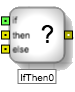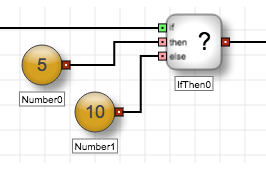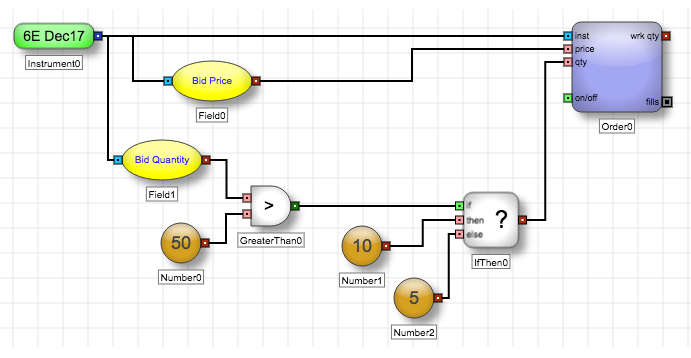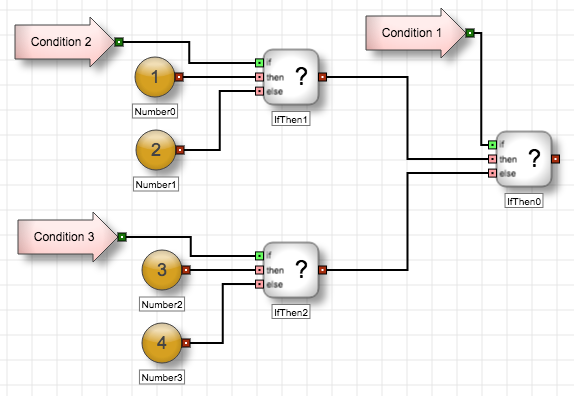Logic blocks

# If Then blockThe If Then block outputs one of two values, based on the value of a Boolean input. When the input value is TRUE, the block outputs a value based on the block's ThenValue condition. When the input value is false, the block outputs a value based on the block's ElseValue condition.

Example The If Then block receives a Boolean value through its 'if' port, then outputs 5 if the value is TRUE or 10 if the value is FALSE.Like the Equal block, the If Then block uses yellow variable input ports, which allow you to specify different types of data (numeric, Boolean, or instrument). Because both inputs must be the same type of data, the block automatically sets the data type for the second input port as soon as you connect the other port. Also, the output port dynamically adjusts to match the data type of the input ports.

Example The following examples uses an If Then block to determine a quantity to use when submitting an order. The If Then block checks the current Bid quantity for the instrument. If the Bid quantity is greater than 50, the If Then block outputs 10 to the Order block's Quantity port. Otherwise, it outputs 5.You can link multiple If Then blocks together to define nested if-then statements as shown in the following example. To find the correct output of nested If Then blocks, always start with the right-most If Then block and trace the logical pathway to the left until arriving at an output.

Example Using nested If Then blocks## Block properties

To customize the block, update the Block Properties.

Property Description
Name Name to display beneath the block on the ADL canvas